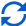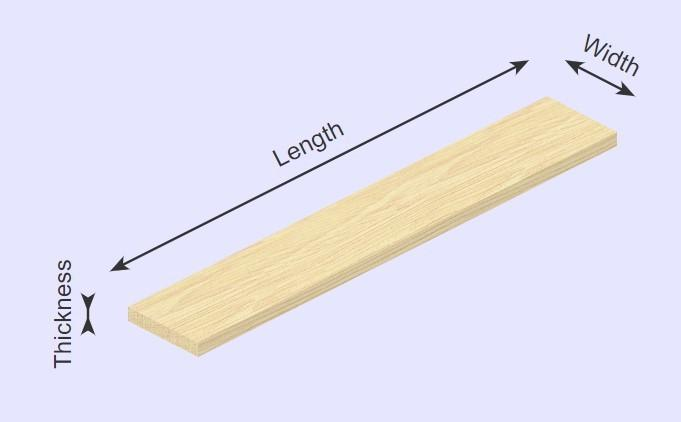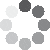Enter parameters
Enter parameters
LENGTH, WIDTH & Thickness
Length:
ft
• inches (in)
• feet (ft)
• yards (yd)
• centimeters (cm)
• meters (m)
Width:
in
• inches (in)
• feet (ft)
• yards (yd)
• centimeters (cm)
• meters (m)
Thickness:
in
• inches (in)
• feet (ft)
• yards (yd)
• centimeters (cm)
• meters (m)
Number of pieces:
Price
per ln.ft
• per Linear Foot (per ln.ft)
• per Board Foot (per bd.ft)Result
Linear feet:
0
Board feet:
0
Cost:
$(1 votes, average: 5.00 out of 5)Loading... Similar Calculators: Real-time graphics. Make the calculations and see the changes. Embed Similar Calculators:You’ll agree with us when we say that working out how much wood your next landscaping project will require is tricky. You want to be as accurate as possible to limit any material waste and in doing so, save yourself some money. That’s why we built our free online lumber calculator. 👍 Keep reading to see just how easy the calculator is to use, what it calculates and how it does it. Contents: ## Lumber calculator formulaOur calculator works out the board feet of standard three dimensional wood material based on the measurements you provide. It does this by using the formula: $$Board\,Feet\,(bf) = {Thickness\,(in) \times Width\,(in) \times Length\,(ft) \over 12}$$ Note: You can give measurements in imperial units (inches, feet, yards) or metric units (centimeters, meters). If you have multiple planks of wood lying end to end, then the calculator can also work out the total board feet of the entire construction: $$Total\,Board\,Feet = Board\,Feet \times Number\,of\,Pieces$$ The calculator also works out the total length of the construction: $$Linear\,Feet = Length \times Number\,of\,Pieces$$ ## How to calculate project cost If you know the price per linear foot or board foot, the calculator can work out the total cost of the wood using the formula: $$Cost = Price\,Per\,Board\,Foot \times Total\,Board\,Feet$$ or $$Cost = Price\,Per\,Linear\,Foot \times Linear\,Feet$$ In addition, you can calculate the price per board foot/linear foot for the wood you have purchased using the formula: $$Price\,Per\,Board\,Foot = {Total\,Cost\,of\,Wood \over Total\,Board\,Feet\,of\,Wood}$$ or $$Price\,Per\,Linear\,Foot = {Total\,Cost\,of\,Wood \over Total\,Linear\,Feet\,of\,Wood}$$ Something not clear? 🤔 See below for two full examples working with different initial criteria. Otherwise, make your measurements accurately and enter the values into our online lumber calculator to get started! ## Example calculations ✔️ Five planks of wood with known price per linear foot Let’s say I need five planks of wood measuring 10 feet in length, 3 inches wide and 1 inch deep. The wood costs$12 per linear foot.

I want to estimate the total board feet and cost for this landscaping project.

I would enter these measurements into the calculator.

It would then perform the following calculations to work out total board feet and linear feet:

$$Board\,Feet\,(bf) = {Thickness\,(in) \times Width\,(in) \times Length\,(ft) \over 12} = {1\,in \times 3\,in \times 10\,ft \over 12} = 2.5\,bf$$

$$Total\,Board\,Feet = Board\,Feet \times Number\,of\,Pieces = 2.5\,bf \times 5 = 12.5\,bf$$

$$Linear\,Feet = Length \times Number\,of\,Pieces = 10\,ft \times 5 = 50\,ft$$

Finally, the calculator would work out the total cost of the wood:

$$Cost = Price\,Per\,Linear\,Foot \times Linear\,Feet = 12\,/ft \times 50\,ft = 600$$

✔️ Two planks of wood calculating price per board foot

For our second example let’s imagine I need to lay down two planks of wood each measuring 12 feet in length, 5 inches wide and 2 inches deep for a construction build.

I don’t know the price per board foot, but I can purchase 20 board feet for a cost of \$60.

I can therefore calculate the price per board foot using the formula:

$$Price\,Per\,Board\,Foot = {Total\,Cost\,of\,Wood \over Total\,Board\,Feet\,of\,Wood} = {60 \over 20\,ft} = 3\,/bf$$

I would then enter the measurements into the calculator to calculate the total board feet and linear feet.

The calculator would perform the following operations:

$$Board\,Feet\,(bf) = {Thickness\,(in) \times Width\,(in) \times Length\,(ft) \over 12} = {2\,in \times 5\,in \times 12\,ft \over 12} = 10\,bf$$

$$Total\,Board\,Feet = Board\,Feet \times Number\,of\,Pieces = 10\,bf \times 2 = 20\,bf$$

$$Linear\,Feet = Length \times Number\,of\,Pieces = 12\,ft \times 2 = 24\,ft$$

Finally, the estimator would work out the total cost of the wood:

$$Cost = Price\,Per\,Board\,Foot \times Total\,Board\,Feet = 3\,/bf \times 20\,bf = 60$$

It’s that simple! 😉

Embed this calculator on your site!Add live graphics
Copied to clipboard! Preview